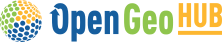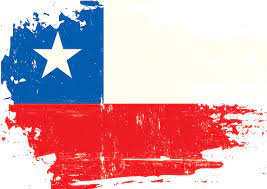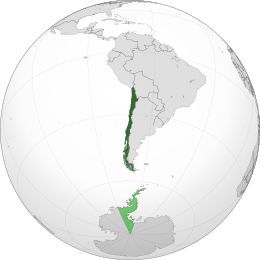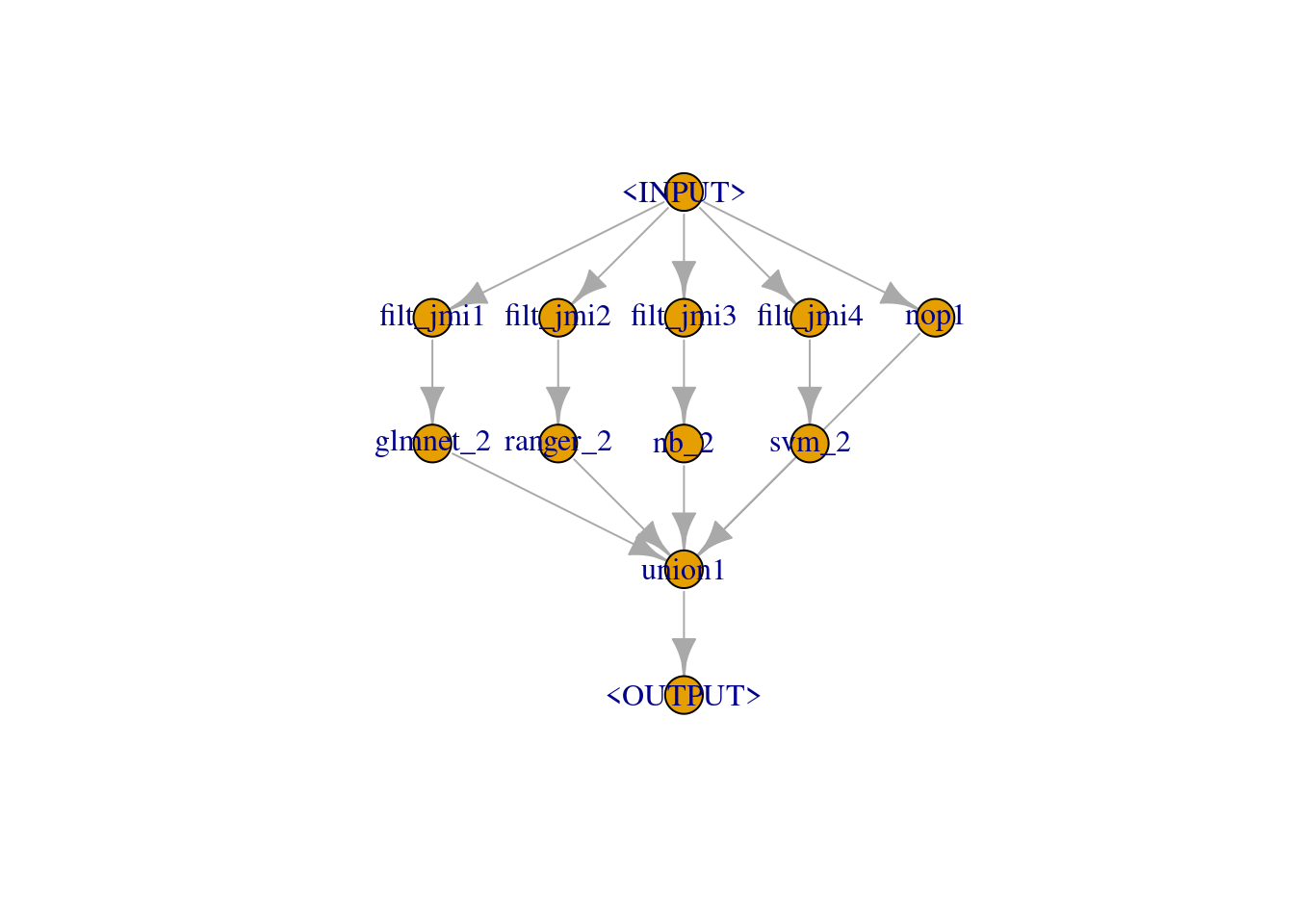# Hackathon Summer School 2022

Classification of pasture areas through EO data and machine learning

Author

Francisco Zambrano (from Chile)# 1 Background

Land use and land cover (LULC) change is a key topic to understand the human footprint and environmental impacts on the planet (Winkler et al., 2019). In recent years the convergence of publicly available Earth Observation (EO) data (Ma, Y. et al., 2015), more accessible cloud-computing platforms and modern machine learning (ML) techniques (Gorelick et al., 2017; Schramm et al., 2021) allowed the production of several LULC maps at national (Souza et al., 2020), continental (Witjes et al., 2022) and global scale (Venter et al., 2022). In general, these products are self-consistent, which means that they map consistently different LULC classes considering distinct training samples and ML techniques, an aspect that makes the comparison across them challenging (Venter et al., 2022). Even so, most of them report a relatively low accuracy for pasture / grasslands classes, a LULC class that suffers from a lack of precise definitions able to incorporate cross-disciplinary evidence of animal production systems into mapping products (Phelps et al., 2017). These areas are well-known to be the single most extensive land cover class of the Earth’s ice-free land surface (Ellis et al., 2010) and highly affected by climate change (Gao et al., 2016). Considering the global relevance of this LULC class WRI, OpenGeoHub, LAPIG and IIASA initiated a Global Pastures Monitoring project supported by the Land & Carbon Lab (LCL) initiative.

# 2 Task definition

The task is to develop a reproducible computation notebook using your favorite programming language (Julia, Python or R) explaining step-by-step the implemented workflow and providing evidence for any claims that you make.

The computation notebook must include at least the following steps (minimum requirements):

1. Download and access the training, validation and test set files publicly available in Parquet and CSV format,
2. Train a machine learning model able to predict the three land cover classes established by the dataset (column class),
3. Predict the test set using the trained model,
4. Upload your predictions in a Google Drive and insert the URL link to access them in this Google Sheet, so we can derive a macro average f1-score (arithmetic mean of f1-score for the three classes, which is insensitive to the imbalance of the classes). IMPORTANT: Remember to change the access of uploaded file to “Anyone with the link”.

# 3 Procedure

To download the data I used the script recommended with the instruction of the hackaton. The data was in parquet format and was read with `arrow::read_parquet` function.

Code
``````library(arrow)

read_pq_from_url <- function(url) {
dst <- basename(url)

if (!file.exists(dst)) {
}

}

train_url <-'https://s3.eu-central-1.wasabisys.com/global-pastures/samples/lcv_pasture_classif.matrix.train_2000..2020_brazil.eumap_summer.school.2022.pq'
val_url <- 'https://s3.eu-central-1.wasabisys.com/global-pastures/samples/lcv_pasture_classif.matrix.val_2000..2020_brazil.eumap_summer.school.2022.pq'
test_url <- 'https://s3.eu-central-1.wasabisys.com/global-pastures/samples/lcv_pasture_classif.matrix.test_2000..2020_brazil.eumap_summer.school.2022.pq'

## 3.2 Merging datasets

The training and validation datasets were merged to create an unifed dataset, then this dataset was spli into `training` and `test`. The prediction data in which I want to run the model will be `new_data`.

Code
``````## 02. Creating additional covariates
library(dplyr)
library(tidyr)

data <- train_data |>
bind_rows(val_data) |>
as_tibble() |>
select(-c(tile_id:longitude,class_pct,class_label)) |>
drop_na() |>
mutate(class = as.factor(class)) |>
janitor::clean_names() |>
select(-index_level_0)

new_data <- test_data |>
as_tibble() |>
select(-c(tile_id:longitude)) |>
drop_na() |>
janitor::clean_names() |>
select(-index_level_0) ``````

## 3.3 Setting seed and a logger

Code
``````set.seed(321)
lgr::get_logger("mlr3")\$set_threshold("warn")
lgr::get_logger("bbotk")\$set_threshold("warn")``````

I created a task with the data merged using the varibale “class” as the target for the model. Then I made a partition of the data using 70% for training and 30% for testing.

## 3.4 Task and partition of train and test dataset

Code
``````library(mlr3)
task <- as_task_classif(data, target = "class",id = 'train')
split <- partition(task,ratio = .7)``````

## 3.5 Learners selected for the classification

First, I created the `task` using the `train_data`, the target is the `class` variable.

I selected the following learners, as the ones that will be stacked for the classification.

1. `glmnet` : Lasso and Elastic-Net Regularized Generalized Linear Models ({glmnet}),
2. `ranger` : A Fast Implementation of Random Forests, ({ranger})
3. `naive_bayes`, Naive Bayes Classifier (`{e1071}`)
4. `svm`: super vector machine (`{e1071}`)
Code
``````library(mlr3learners)
lrn_glmnet = lrn("classif.glmnet", predict_type = "prob")
lrn_ranger = lrn("classif.ranger", predict_type = "prob")
lrn_nb = lrn("classif.naive_bayes",predict_type = "prob")
lrn_svm = lrn("classif.svm", predict_type = "prob")``````

## 3.6 Pipeline {mlr3pipelines}

To train the stacked models I used `{mlr3pipelines}` by layers. The layers were:

1. `level_0` : feature selection using `{mlr3filters}` and out-of-bag predictions for the learners
2. `level_1` : principal components analysis (PCA) and out-of-bag predictions for the learners
3. `level_2` : random forest with `{ranger}`

### 3.6.1 level_0

Code
``````library(mlr3pipelines)

cv2_glmnet = po("learner_cv", lrn_glmnet, id = "glmnet_2")
cv2_ranger = po("learner_cv", lrn_ranger, id = "ranger_2")
cv2_nb = po("learner_cv", lrn_nb, id = "nb_2")
cv2_svm = po("learner_cv", lrn_svm, id = "svm_2")

# 07.- Filters for feature selection
library(mlr3filters)
jmi1 = po("filter", flt("jmi"), id = "filt_jmi1",filter.nfeat =2)
jmi2 = po("filter", flt("jmi"), id = "filt_jmi2",filter.nfeat =2)
jmi3 = po("filter", flt("jmi"), id = "filt_jmi3",filter.nfeat =2)
jmi4 = po("filter", flt("jmi"), id = "filt_jmi4",filter.nfeat =2)

# 08.- union for level0

level_0 = gunion(list(
jmi1 %>>% cv2_glmnet,
jmi2 %>>% cv2_ranger,
jmi3 %>>% cv2_nb,
jmi4 %>>% cv2_svm,
po("nop", id = "nop1")))  %>>%
po("featureunion", id = "union1")

level_0\$plot()``````### 3.6.2 level_1

Code
``````cv3_glmnet = po("learner_cv", lrn_glmnet, id = "glmnet_3")
cv3_ranger = po("learner_cv", lrn_ranger, id = "ranger_3")
cv3_nb = po("learner_cv", lrn_nb, id = "nb_3")
cv3_svm = po("learner_cv", lrn_svm, id = "svm_3")

level_1 = level_0 %>>%
po("copy", 5) %>>%
gunion(list(
po("pca", id = "pca_1", param_vals = list(scale. = TRUE)) %>>% cv3_glmnet,
po("pca", id = "pca_2", param_vals = list(scale. = TRUE)) %>>% cv3_ranger,
po("pca", id = "pca_3", param_vals = list(scale. = TRUE)) %>>% cv3_nb,
po("pca", id = "pca_4", param_vals = list(scale. = TRUE)) %>>% cv3_svm,
po("nop", id = "nop3")
)) %>>%
po("featureunion", id = "union2")

level_1\$plot()``````